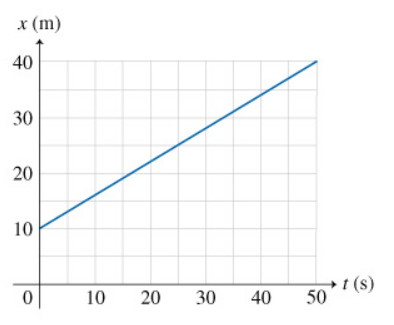# Problem: What is the average velocity vav of the particle over the time interval Δt=50.0 s ?

###### FREE Expert Solution

The average velocity, vavg of the particle is given by the change in position divided by the change in time.

vavg = Δx/Δt###### Problem Details

What is the average velocity vav of the particle over the time interval Δt=50.0 s ?